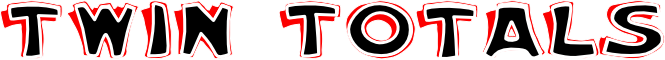#Which two of the following calculations give the same total?

 80 + 29 1 + 177 60 + 23 33 - 20 86 + 75 104 + 63 77 + 20 30 - 13 10 x 10 67 + 113 104 + 74 9 x 5 45 + 10 75 + 39 40 + 56 20 + 11 32 - 20 10 x 4 1 x 1 21 + 56

## A Mathematics Lesson Starter Of The Day

Topics: Starter | Arithmetic

• 7abm2, King Alfred's College, Oxfordshire UK
•
• We enjoyed the starter and we got the correct answer we thought that it was very easy! :).
• Cobham Intermediate, R22 Maths
•
• Too easy, need more of a challenge,
Rate 2.

[Transum: Thanks for your comments. Buttons have been added below to give you the option of larger numbers.]
• Elen Catherine, Ysgol Pen Y Bryn
•
• I love Maths and Starter of the day.

How did you use this starter? Can you suggest how teachers could present or develop this resource? Do you have any comments? It is always useful to receive feedback and helps make this free resource even more useful for Maths teachers anywhere in the world.

Previous Day | This starter is for 21 June | Next Day

 80 + 29 = 109 1 + 177 = 178 60 + 23 = 83 33 - 20 = 13 86 + 75 = 161 104 + 63 = 167 77 + 20 = 97 30 - 13 = 17 10 x 10 = 100 67 + 113 = 180 104 + 74 = 178 9 x 5 = 45 45 + 10 = 55 75 + 39 = 114 40 + 56 = 96 20 + 11 = 31 32 - 20 = 12 10 x 4 = 40 1 x 1 = 1 21 + 56 = 77

Note to teacher: Doing this activity once with a class helps students develop strategies. It is only when they do this activity a second time that they will have the opportunity to practise those strategies. That is when the learning is consolidated. Click the button above to regenerate another version of this starter from random numbers.

Your access to the majority of the Transum resources continues to be free but you can help support the continued growth of the website by doing your Amazon shopping using the links on this page. Below is an Amazon search box and some items I have chosen and recommend to get you started. As an Amazon Associate I earn a small amount from qualifying purchases which helps pay for the upkeep of this website.Teacher, do your students have access to computers?Do they have iPads or Laptops in Lessons? Whether your students each have a TabletPC, a Surface or a Mac, this activity lends itself to eLearning (Engaged Learning).Transum.org/go/?Start=June21

Here is the URL which will take them to a related student activity.

Transum.org/go/?to=chainFor All: